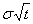# Options

Option is a derivative financial instrument. This means, not only the option itself, also something under it, is a part of the contract. This something, we call it underlying instrument, can be for example stock, bond, swap, amount in foreign currency, stock or bond index, gold, copper, cattle meat or even a nice weather.

There are two kinds of options: call and put. Exact definition is:

The buyer of a call option buys a right (but no obligation) to buy later the underlying instrument (from the seller of the option) for a fixed price (the strike of the option).
The buyer of a put option buys a right (but no obligation) to sell later the underlying instrument (to the seller of the option) for a fixed price (the strike of the option).

For this right, the buyer of the option has to pay to the seller premium in advance: the price of the option. When the buyer of the option exercises his right, we speak about exercise of the option. By exercise one can agree either upon physical delivery or cash settlement. Physical delivery and e.g. call option means, the buyer of the option pays the strike and get the underlying instrument. Cash settlement means, the seller of the option has to pay to the buyer the price difference between price of the underlying and strike. Options do not last forever, (otherwise they were too expensive). They have a maturity. If they are not exercised until maturity, they expire worthless.

## Option price

In order to determine a price or a value of the option, we need at least the following specifications:

• Value Day: The day on which the premium has to be paid, or the day we value the option,
• Current price of an underlying: Underlying Price or its fixed future price: Underlying Forward Price,
• Maturity
• Discount Interest Rate until maturity,
• Strike and
• Fluctuation of the underlying price (standard deviation).

The longer the maturity, the greater the fluctuation of an underlying price. We assume that the fluctuation grows with the square root of time. The standard deviation of an underlying price for the time of exactly one year we call the volatility of the underlying price. The standard deviation of the underlying price is then, where σ is the volatility and t is the time to maturity in years.

## Sensitivities of the Option Price (Greeks)

Not only the price of the option is interesting but also how the price changes if we change some of price building components. The most important sensitivity is Delta: the partial derivative of an option to the price of an underlying. Delta=0.5 means, if the price of an underlying changes to a (very small) amount, the price of the option changes only to a half of this amount. Other sensitivities are:

• Gamma is partial derivative of Delta to the underlying price.
• Theta is partial derivative of option price to maturity. We show how the price changes if maturity is one day shorter.
• Rho is partial derivative of option price to discount interest rate. We show how the price changes if the discount interest rate grows 1 percent.
• Vega is partial derivative of option price to volatility. We show how much the price changes if volatility grows at 0.01.
• Kappa is partial derivative of option price to strike.

## Classification of Options

We can classify options on the underlying type, on kind of exercise and on exercise amount. By classification on the underlying type we can distinguish between:

• Options on stock or equity index: Equity and Equity Index Options
• Options on foreign exchange: FX Options
• Options on interest rate: Caplets, Floorlets, Caps and Floors
• Options on bond or swap: Bond Options or Swaptions
• Options on commodity or commodity index: Commodity Options
• Options on futures
• Options on more than one underlying: Basket Options
• Options on options

By classification on kind of exercise we have:

• European Options can be exercised only on maturity.
• American Options can be exercised all the time until maturity.
• Bermuda Options can be exerciesed only on some previously determined days until maturity.
• Barrier Options may only be exercised on the maturity if the underlying price crossed (=Knock In) resp. did not cross (=Knock Out) some barrier until maturity.
• Double Barrier Options are barrier options with two barriers.

By exercise amount we can divide between:

• By plain (Plain-Vanilla) options exercise amount is a difference between underlying price and strike price.
• By Digital Options exercise amount is fixed (money) amount. Options may only be exercised if on maturity the underlying price is higher (Call Option) resp. lower (Put Option) then the strike.
• By Asian Options exercise amount is the difference between average price of underlying and strike price. The average underlying price is build up from underlying prices on some previously determined days. Sometimes, the exercise amount is the difference between the average price and final price of underlying on maturity (Average Strike Asian Options).
• By Lookback Options, exercise amount is the difference between the highest and final underlying price (Call Option) resp. the difference between final and lowest underlying price (Put Option).
• By Chooser Options the buyer of an option can choose (after some time) if she wants a Call- or a Put-Option.